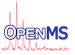OpenMS  2.8.0
TransformationModelInterpolated Class Reference

Interpolation model for transformations. More...

`#include <OpenMS/ANALYSIS/MAPMATCHING/TransformationModelInterpolated.h>`

Inheritance diagram for TransformationModelInterpolated:
[legend]
Collaboration diagram for TransformationModelInterpolated:
[legend]

## Classes

class  Interpolator
The class defines a generic interpolation technique used in the TransformationModelInterpolated. More...

## Public Member Functions

TransformationModelInterpolated (const DataPoints &data, const Param &params)
Constructor. More...

TransformationModelInterpolated (const std::vector< std::pair< double, double >> &data, const Param &params, bool preprocess)

~TransformationModelInterpolated () override
Destructor. More...

double evaluate (double value) const override
Evaluate the interpolation model at the given value. More...Public Member Functions inherited from TransformationModel
TransformationModel ()
Constructor. More...

TransformationModel (const TransformationModel::DataPoints &, const Param &)

virtual ~TransformationModel ()
Destructor. More...

virtual void weightData (DataPoints &data)
Weight the data by the given weight function. More...

virtual void unWeightData (DataPoints &data)
Unweight the data by the given weight function. More...

bool checkValidWeight (const String &weight, const std::vector< String > &valid_weights) const
Check for a valid weighting function string. More...

double checkDatumRange (const double &datum, const double &datum_min, const double &datum_max)
Check that the datum is within the valid min and max bounds. More...

double weightDatum (const double &datum, const String &weight) const
Weight the data according to the weighting function. More...

double unWeightDatum (const double &datum, const String &weight) const
Apply the reverse of the weighting function to the data. More...

const ParamgetParameters () const
Gets the (actual) parameters. More...

std::vector< StringgetValidXWeights () const
Returns a list of valid x weight function strings. More...

std::vector< StringgetValidYWeights () const
Returns a list of valid y weight function strings. More...

## Static Public Member Functions

static void getDefaultParameters (Param &params)
Gets the default parameters. More...Static Public Member Functions inherited from TransformationModel
static void getDefaultParameters (Param &params)
Gets the default parameters. More...

## Private Member Functions

void preprocessDataPoints_ (const DataPoints &data)
Preprocesses the incoming data and fills the (private) vectors x_ and y_. More...

void preprocessDataPoints_ (const std::vector< std::pair< double, double >> &data)
Preprocesses the incoming data and fills the (private) vectors x_ and y_. More...

## Private Attributes

std::vector< double > x_
Data coordinates x. More...

std::vector< double > y_
Data coordinates y. More...

Interpolatorinterp_
Interpolation function. More...

TransformationModelLinearlm_front_
Linear model for extrapolation (front) More...

TransformationModelLinearlm_back_
Linear model for extrapolation (back) More...Public Types inherited from TransformationModel
typedef std::vector< DataPointDataPoints
Vector of coordinate pairs. More...Protected Attributes inherited from TransformationModel
Param params_
Parameters. More...

String x_weight_
x weighting More...

double x_datum_min_

double x_datum_max_

String y_weight_
y weighting More...

double y_datum_min_

double y_datum_max_

bool weighting_

## Detailed Description

Interpolation model for transformations.

Between the data points, the interpolation uses the neighboring points to interpolate. The following interpolation methods are available:

• linear: Linearly interpolate between neighboring points
• cspline: Use a cubic spline to interpolate between neighboring points
• akima: Use an akima spline to interpolate between neighboring points (less affected by outliers)

Outside the range spanned by the points, we extrapolate using one of the following methods:

• two-point-linear: Uses a line through the first and last point to extrapolate
• four-point-linear: Uses a line through the first and second point to extrapolate in front and and a line through the last and second-to-last point in the end. If the data is non-linear, this may yield better approximations for extrapolation.
• global-linear: Uses a linear regression to fit a line through all data points and use it for extrapolation. Note that global-linear extrapolation may not be continuous with the interpolation at the border.

## ◆ TransformationModelInterpolated() [1/2]

 TransformationModelInterpolated ( const DataPoints & data, const Param & params )

Constructor.

Parameters
 data The known data points. params Param object holding information on which model to choose.
Exceptions
 IllegalArgument is thrown if there are not enough data points or if an unknown interpolation type is given.

## ◆ TransformationModelInterpolated() [2/2]

 TransformationModelInterpolated ( const std::vector< std::pair< double, double >> & data, const Param & params, bool preprocess )

## ◆ ~TransformationModelInterpolated()

 ~TransformationModelInterpolated ( )
override

Destructor.

## ◆ evaluate()

 double evaluate ( double value ) const
overridevirtual

Evaluate the interpolation model at the given value.

Parameters
 value The position where the interpolation should be evaluated.
Returns
The interpolated value.

Reimplemented from TransformationModel.

## ◆ getDefaultParameters()

 static void getDefaultParameters ( Param & params )
static

Gets the default parameters.

## ◆ preprocessDataPoints_() [1/2]

 void preprocessDataPoints_ ( const DataPoints & data )
private

Preprocesses the incoming data and fills the (private) vectors x_ and y_.

## ◆ preprocessDataPoints_() [2/2]

 void preprocessDataPoints_ ( const std::vector< std::pair< double, double >> & data )
private

Preprocesses the incoming data and fills the (private) vectors x_ and y_.

## ◆ interp_

 Interpolator* interp_
private

Interpolation function.

## ◆ lm_back_

 TransformationModelLinear* lm_back_
private

Linear model for extrapolation (back)

## ◆ lm_front_

 TransformationModelLinear* lm_front_
private

Linear model for extrapolation (front)

## ◆ x_

 std::vector x_
private

Data coordinates x.

## ◆ y_

 std::vector y_
private

Data coordinates y.